# 06-1350/Homework Assignment 3

Solve the following problem and submit your solution in class by November 16, 2006:

Problem. The product of two polynomials is again a polynomial; there must be an analog for that in the world of "polynomial" invariants of knots.

1. Prove that the product of two finite type invariants (of, say, knotted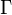$\Gamma$'s) is again a finite type invariant. Of what type will it be, as a function of the types of the two factors of the product?
2. In what way does the product of finite type invariant induces a map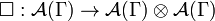$\Box:{\mathcal A}(\Gamma)\to{\mathcal A}(\Gamma)\otimes{\mathcal A}(\Gamma)$?
3. Describe the map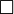$\Box$ of above in explicit terms. First use the "chords and 4T" description of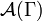${\mathcal A}(\Gamma)$, and then the "trivalent diagrams and AS, IHX and STU" description of the same object.
4. Learn somewhere about coalgebras and show that$\Box$ is always coassociative and cocommutative.
5. Learn somewhere about bialgebras (Hopf algebras without an antipode) and show that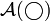${\mathcal A}(\bigcirc)$ becomes a commutative associative cocommutative and coassociative bialgebra, if taken with the "connected sum" product and with$\Box$ as a coproduct.

With a tiny bit of further algebra and quoting an old theorem of Milnor and Moore, it follows that${\mathcal A}(\bigcirc)$ is a commutative graded polynomial algebra with finitely many generators at each degree. The dimension of the spaces of generators at degrees up two 12 are known and are denoted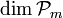$\dim{\mathcal P}_m$ in the table below, which is reproduced from 06-1350/Class Notes for Thursday October 12:

 m 0 1 2 3 4 5 6 7 8 9 10 11 12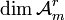$\dim{\mathcal A}_m^r$ 1 0 1 1 3 4 9 14 27 44 80 132 232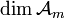$\dim{\mathcal A}_m$ 1 1 2 3 6 10 19 33 60 104 184 316 548$\dim{\mathcal P}_m$ 0 1 1 1 2 3 5 8 12 18 27 39 55